# Which has better sensitivity KNN or decision tree?

Which has better sensitivity KNN or decision tree?

Let’s investigate two machine learning algorithms: K-Nearest Neighbors (KNN) and Decision Tree. Hepatitis dataset and Diabetic Retinopathy Debrecen dataset1 were used to train the models and to test their accuracy in classifying the test instances. Data pre-processing including data cleaning, feature selection and normalization was performed. Different values of hyper-parameter K and different methods to measure distance were tried to find the optimal KNN model. A variety of tree depths and cost functions were also examined when studying the Decision Tree algorithm.

For Hepatitis dataset, KNN algorithm with K = 3, using Manhattan distance and ASCITES and PROTIME as features is optimal. When applying Decision Tree on Hepatitis dataset, a tree with a maximum depth of 3, using misclassification cost and the same two features gives the best performance. For Diabetic Retinopathy Debrecen dataset, the optimal KNN model takes K = 7, Manhattan distance and all the features. Furthermore, the best tree chooses a maximum depth of 10, Entropy cost and all the features. For both datasets, the accuracy of KNN is slightly higher than that of Decision Tree.

#### 2 Introduction

KNN and Decision Tree are two commonly used non-parametric machine learning algorithms for regression and classification. KNN algorithm is a lazy learner and does nothing but stores the data during training. It looks for K-nearest neighbors to predict the labels of test instances. Different choices of K values and ways to define distance/similarity can affect the accuracy of KNN algorithms. Decision Tree divides the training data into regions where the cost is minimized through successively splitting regions according to one variable each time. The performance of Decision Tree depends on maximum tree depth4 and the choice of cost function5. In this project, we aimed to compare the performance of KNN and Decision Tree on classification problems.

##### We would also like to discover the optimal K and distance function for KNN and the best tree depth and cost function for Decision Tree. Moreover, the effects of feature selection and normalization were studied as they are of great importance in reducing model complexity and increasing accuracy according to previous studies.6

Two datasets from UCI Irvine Machine Learning Repository1 were used to train and test the models. The first is called Hepatitis dataset, which includes 155 instances and 19 features describing the physical conditions and symptoms of Hepatitis patients. 75 out of 155 instances were found to contain missing values. As a result, for the Hepatitis dataset, the goal is to predict whether a person will die of Hepatitis C, a liver disease.7 In addition, the second dataset Diabetic Retinopathy Debrecen dataset records 1151 instances, none of which has missing values. Lastly, there are 19 features in the Diabetic Retinopathy Debrecen dataset, which describe the eye fundus images and can be used to predict whether a sign of Diabetic Retinopathy (DR) exists.

##### We found that the choices of hyper-parameters, cost/distance functions and features all had an impact on the accuracy of KNN and Decision Tree.

And the optimal model for one dataset might not be suitable for others. When applying KNN and Decision Tree to Hepatitis dataset, the model reaches the highest accuracy at K = 3 and maximum tree depth = 3 respectively. Manhattan distance and misclassification cost are used in the optimal KNN and Decision Tree models. For both algorithms, the models using ASCITES and PROTIME as predictors have the best performance. The effect of normalization on model performance for both algorithms is inconsistent across different feature choices. For Diabetic Retinopathy Debrecen dataset, K = 7 and maximum tree depth of 10 give the highest accuracy. Manhattan distance is still chosen for KNN but entropy cost becomes the optimal cost function for Decision Tree. All the features in the dataset should be used. Normalization in these cases has almost no effects on the accuracy of prediction.

For both datasets, KNN has a greater accuracy than Decision Tree. However, applying either method, the prediction accuracy on Diabetic Retinopathy Debrecen dataset is significantly lower than that of the Hepatitis dataset. This may be due to the low correlation between the features and class in Diabetic Retinopathy Debrecen dataset.

#### 3 Datasets

There are two datasets used in this project: Hepatitis dataset and Diabetic Retinopathy Debrecen dataset. The Hepatitis dataset contains 19 features about the physical and medical conditions of 155 Hepatitis C patients and classifies the individuals based on whether the individual dies of Hepatitis. Diabetic Retinopathy Debrecen dataset includes 19 features describing the eye fundus images of 1151 patients and puts individuals into classes based on whether they have signs of DR.

Firstly we examined whether the dataset contained any missing values. We found that 75 out of 155 instances in the Hepatitis dataset had missing values while there was no lost information in the other dataset. The problem was solved by simply removing any rows with missing values.

Then we investigated the distribution of the features and classes of the two datasets. The distribution plots are shown below. The correlations between these features were also calculated.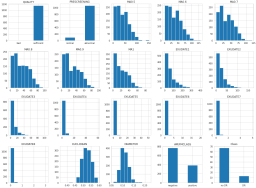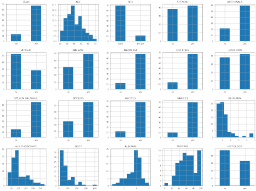###### The distributions of the attributes in Hepatitis dataset. The distributions of the attributes in the DR dataset.

We decided to drop or merge features having mutual correlations > 0.4 to create a reduced model. In the Hepatitis dataset, MALAISE and ALBUMIN were dropped due to their high correlations with FATIGUE and ASCITES respectively. The other two correlated features LIVER BIG and LIVER FIRM were merged by taking average. In Diabetic Retinopathy Debrecen dataset, MA0.5 to MA1, EXUDATE1 to EXUDATE4 and EXUDATE4 to EXUDATE were merged separately by taking averages. Furthermore, ASCITES and PROTIME in Hepatitis dataset and MA (average of MA0.5 to 1) and EXUDATE4-8 (average of EXUDATE4 to 8) in Diabetic Retinopathy Debrecen dataset were selected to form models of two features to test the performance of reduced models, because the two pairs of features are most correlated with Class in their own dataset.

##### One main issue the two datasets may cause is that they have very unbalanced distributions of Class. In the Hepatitis dataset, we have almost 5 instances in LIVE class compared to DIE. Similar situation occurs in the other dataset. This can bias the prediction towards the majority class.

Regarding ethical concerns, when dealing with this kind of healthcare data involving patients’ personal medical records, it is important to avoid improper use or unnecessary spread of the information in the datasets.

#### 4 Results

In this section, we first investigated and decided the best parameters for KNN and Decision Tree using 10-fold cross validation. Then, we presented the performance of the two machine learning algorithms on Hepatitis dataset and Diabetic Retinopathy Debrecen dataset. The last subsection demonstrates plots of decision boundaries of the predictive models for the two datasets. In order to compare the performance of KNN and Decision Tree accurately, 20 iterations were run with different training sets and testing sets each time for the experiments in Section 4.1.2. For each iteration, we randomly selected 80% of data as the training set and the rest as the testing set. We evaluated the performance of the predictive models using the metric accuracy.

#### 4.1 Comparison of KNN and Decision Tree with Different Parameters 4.1.1 Cross Validation for choosing K and max depth

Firstly, we used 10-fold cross validation to find the best parameters, the number of neighbors K in KNN and the max depth in the Decision Tree for both datasets respectively. The cross validation results (the accuracy of predictions on validation sets) of different parameters for the two datasets are shown in the following figures.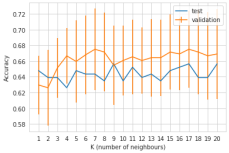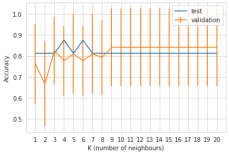###### Accuracy of CV for K on Hepatitis Accuracy of CV for K on Diabetic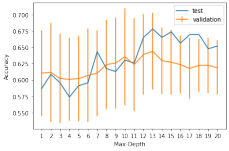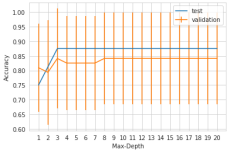###### Accuracy of CV for max-depth on Hepatitis Accuracy of CV for max-depth on Diabetic

Based on the figures, K = 3 was chosen as the parameter of the KNN model for the Hepatitis dataset since the accuracy increases a lot from K = 2 to K = 3. Even though the KNN model can get a higher accuracy for K = 9, there is no large increase between the accuracy at K = 3 and K = 9. Thus, we chose K = 3 for the Hepatitis dataset. Similarly, K = 7 was chosen for the Diabetic dataset as the KNN model gets the highest accuracy using this parameter.

Moreover, 3 was set as the max depth of Decision Tree for the Hepatitis dataset since that model gets the best performance as demonstrated in the figure. For Diabetic dataset, even though the model reaches the highest accuracy with max depth = 13, the accuracy of the validation set does not differ very much (less than 1%) for max depth = 10 and 13. Thus, we chose 10 as the max depth of the Decision Tree model for this dataset. The following experiments all used the selected parameters as described above (K=3, max depth=3 for Hepatitis dataset, and K=7, max depth=10 for Diabetic dataset)

#### 4.1.2 Performance of Classification Models with Different Criteria

Moreover, the performance of KNN and Decision Tree on testing sets are shown in the following tables, both KNN and Decision Tree models were implemented in three conditions, at First, all features in the datasets were used as the input of the predictive models. Then, we used the reduced features with deletion and combination from the data-preprocessing as the input. At last, according to the correlation between features and labels, we selected 2 features for each dataset and used the two selected features as the input data for KNN and Decision Tree models. Moreover, we compared the accuracy of predictive models with raw input data and normalized input data. Lastly, we normalized the input data using mean and standard deviation of each feature.

##### We also tested different distance functions, euclidean and manhattan, for KNN models and different cost functions, misclassification, entropy, and gini-index, for Decision Tree models as shown in the tables.

Thus, for Hepatitis dataset, as shown in the first table, KNN with manhattan function and 2 selected features as input performed the best with an accuracy of 0.89, and Decision Tree with misclassification as the cost function using the 2 selected features as input got an accuracy of 0.85. Moreover, KNN models with euclidean function and models with manhattan function have similar performance. Furthermore, Decision Tree models with different cost functions have similar accuracy on average as well. Overall, the performance of KNN is better than that of Decision Tree, and models using 2 selected features as input data performed better than models using all features and reduced features with deletion and combination as input.

Normalization based on this dataset generally has an improving effect on model accuracy when more features such as all features and reduced features come into play. Except for the cases of Decision Tree algorithm with entropy as cost function using all features in addition, Decision Tree algorithm with gini index as cost function using reduced features. In contrast, normalization has a negative impact on accuracy when we employ only 2 features, so normalization has a mixed effect on the Hepatitis dataset.

#### Accuracy for Models on Diabetic Dataset

For Diabetic dataset, KNN with manhattan distance and all features as input outperformed other models with an accuracy of 0.66, while the best Decision Tree model got an accuracy of 0.63 with entropy function as the hyper-parameter and all features as input. There is no big difference in the performance of KNN models with different distance functions, and Decision Tree with different cost functions also have similar performance. KNN and Decision Tree models with all features as input perform much better than those using other features as input, and models using normalized input have similar or sometimes worse performance than models using raw input.

#### 4.2 Decision Boundary for the 2 Datasets

The following 4 figures show the decision boundary of KNN and Decision Tree models on both datasets respectively. We used the models with the best performance in the last subsection to draw the decision boundary plots. Since we selected all features as the input for models on Diabetic Dataset, according to the correlation between features, we chose 2 key features, ”MA0.5” and ”EUCLIDEAN”, as the features shown in the plots.

###### Decision boundary of KNN on Hepatitis Decision boundary of KNN on Diabetic : Which has better sensitivity KNN or decision tree?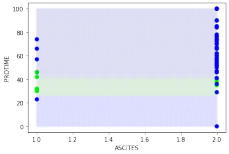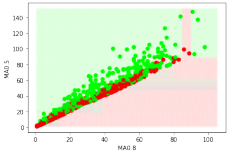#### 5 Discussion and Conclusion

In this project, we visualized and analyzed data distribution and finished data preprocessing based on correlations between features for both Hepatitis dataset and Diabetic Retinopathy Debrecen dataset. Moreover, we investigated different settings of hyper-parameters and different input features for both K-Nearest Neighbor (KNN) and Decision Tree models and evaluated the performance of models using the metric accuracy. 10-fold cross validation, we used for choosing the parameter K in KNN and max depth in the Decision Tree. Furthermore, for both dataset, KNN models outperformed Decision Tree models. The method that we used to select features worked better on Hepatitis dataset, and models using the 2 selected features as input data on this dataset performed the best.

For the future work, we would like to implement K-Nearest Neighbor and Decision Tree models and compare with other baseline models, such as Support Vector Machine (SVM) and Multilayer Perceptron (MLP), on other classification problems to explore the performance of the two models and the effect of hyper-parameters setting for predictive models.

#### Which has better sensitivity KNN or decision tree?References

 Dua, D. and Graff, C. (2019). UCI Machine Learning Repository [http://archive.ics.uci.edu/ml]. Irvine, CA: University of California, School of Information and Computer Science.

 k value Prayudi, I. (2019). What Affects K Value Selection In K-Nearest Neighbor . INTERNATIONAL JOURNAL OF SCIENTIFIC amp; TECHNOLOGY RESEARCH, 8(07).

 Abu Al Failat, H. A., Hassanat, A. B. A., Lasassmeh, O., Tarawneh, A. S., Alhasanat, M. B., Eyal Salman, H. S., amp; Prasath, V. B. S. (2019). Effects of distance measure choice on k-nearest neighbor lastly Classifier Performance: A Review. Big Data, 7(4), 221–248. https://doi.org/10.1089/big.2018.0175

 Bertsimas, D., amp; Dunn, J. (2017). Optimal classification trees. Machine Learning, 106(7), 1039–1082. https://doi.org/10.1007/s10994-017-5633-9

 Zhao, X., amp; Nie, X. (2021). Splitting choice in addition computational complexity analysis of Decision Trees. Entropy, 23(10), 1241. https://doi.org/10.3390/e23101241

 Chen, R.-C., Dewi, C., Huang, S.-W., amp; Caraka, R. E. (2020). Selecting critical features for data classification based on machine learning methods. Journal of Big Data, 7(1). https://doi.org/10.1186/s40537-020- 00327-4

 Bhargav, K. S., & Kumari, T. D. (2018). Application of Machine Learning Classification Algorithm on Hepatitis Dataset. International Journal of Applied Engineering Research, 13(16), 12732–12737.

Which has better sensitivity KNN or decision tree?